## How to Calculate and Solve for Vertical Strain | Rock Mechanics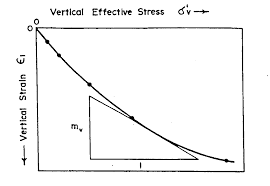The image above represents vertical strain.

To compute for vertical strain, five essential parameters are needed and these parameters are Vertical Stress (σv), Poisson’s Ratio (v), Principal Horizontal Stress Component 1 (σH1), Principal Horizontal Stress Component 2 (σH2) and Young’s Modulus (E).

The formula for calculating the vertical strain:

εv = v – vσH1 – vσH2) / E

Where:

εv = Vertical Strain
σv = Vertical Stress
v = Poisson’s Ratio
σH1 = Principal Horizontal Stress Component 1
σH2 = Principal Horizontal Stress Component 2
E = Young’s Modulus

Let’s solve an example;
Find the vertical strain with a vertical stress of 44, Poisson’s ratio of 5, principal horizontal stress component 1 of 6, principal horizontal stress component 2 of 4 and young’s modulus of 12.

This implies that;

σv = Vertical Stress = 44
v = Poisson’s Ratio = 5
σH1 = Principal Horizontal Stress Component 1 = 6
σH2 = Principal Horizontal Stress Component 2 = 4
E = Young’s Modulus = 12

εv = v – vσH1 – vσH2) / E
εv = (44 – 5(6) – 5(4)) / 12
εv = (44 – 30 – 20) / 12
εv = -6 / 12
εv = -0.5

Therefore, the vertical strain is -0.5.

Calculating the Vertical Stress when the Vertical Strain, Poisson’s Ratio, Principal Horizontal Stress Component 1, Principal Horizontal Stress Component 2 and Young Modulus is Given.

σv = (εv x E) + vσH1 + vσH2

Where:

σv = Vertical Stress
εv = Vertical Strain
v = Poisson’s Ratio
σH1 = Principal Horizontal Stress Component 1
σH2 = Principal Horizontal Stress Component 2
E = Young’s Modulus

Let’s solve an example;
Find the vertical stress with a vertical strain of 52, Poisson’s ratio of 12, principal horizontal stress component 1 of 15, principal horizontal stress component 2 of 10 and young’s modulus of 13.

This implies that;

εv = Vertical Strain = 52
v = Poisson’s Ratio = 12
σH1 = Principal Horizontal Stress Component 1 = 15
σH2 = Principal Horizontal Stress Component 2 = 10
E = Young’s Modulus = 13

σv = (εv x E) + vσH1 + vσH2
σv = (52 x 13) + 12(15) + 15(10)
σv = 676 + 180 + 150
σv = 1006

Therefore, the vertical stress is 1006.

Calculating the Poisson’s Ratio when the Vertical Strain, Vertical Stress, Principal Horizontal Stress Component 1, Principal Horizontal Stress Component 2 and Young Modulus is Given.

v = σv – (εv x E) / σH1 + σH2

Where:

v = Poisson’s Ratio
εv = Vertical Strain
σv = Vertical Stress
σH1 = Principal Horizontal Stress Component 1
σH2 = Principal Horizontal Stress Component 2
E = Young’s Modulus

Let’s solve an example;
Find the Poisson’s ratio with a vertical strain of 16, vertical stress of 110, principal horizontal stress component 1 of 7, principal horizontal stress component 2 of 13 and young’s modulus of 6.

This implies that;

εv = Vertical Strain = 16
σv = Vertical Stress = 110
σH1 = Principal Horizontal Stress Component 1 = 7
σH2 = Principal Horizontal Stress Component 2 = 13
E = Young’s Modulus = 6

v = σv – (εv x E) / σH1 + σH2
v = 110 – (16 x 6) / 7 + 13
v = 110 – 96 / 20
v = 14 / 20
v = 0.7

Therefore, the Poisson’s ratio is 0.7.

## How to Calculate and Solve for Horizontal Stress | Rock Mechanics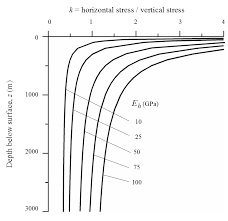The image above represents horizontal stress.

To compute for horizontal stress, two essential parameters are needed and these parameters are Poisson’s ratio (v) and vertical stress (σv).

The formula for calculating the horizontal stress:

σH = v / 1 – v

Where;

σH = Horizontal Stress
v = Poisson’s Ratio
σv = Vertical Stress

Let’s solve an example;
Find the horizontal stress when the Poisson’s ratio is 24 and the vertical stress is 28.

This implies that;

v = Poisson’s Ratio = 24
σv = Vertical Stress = 28

σH = v / 1 – v
σH = 24(28) / (1 – 24)
σH = 672 / -23
σH = -29.217

Therefore, the horizontal stress is 29.217.

Calculating the Poisson’s Ratio when the Horizontal Stress and Vertical stress is Given.

v = σH / σv + σH

Where;

v = Poisson’s Ratio
σH = Horizontal Stress
σv = Vertical Stress

Let’s solve an example;
Find the Poisson’s ratio when the horizontal stress is 12 and the vertical stress is 16.

This implies that;

σH = Horizontal Stress = 12
σv = Vertical Stress = 16

v = σH / σv + σH
v = 12 / 16 + 12
v = 12 / 28
v = 12 / 28
v = 0.428

Therefore, the Poisson’s ratio is 0.428.

## How to Calculate and Solve for Lateral Strain | Rock Mechanics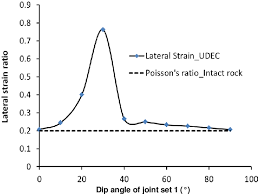The image above represents lateral strain.

To compute for lateral strain, two essential parameters are needed and these parameters are change in diameter (Δd) and diameter (d).

The formula for calculating the lateral strain:

εl = Δd / d

Where:

εl = Lateral Strain
Δd = Change in Diameter
d = Diameter

Let’s solve an example;
Find the lateral strain when the change in diameter is 32 and the diameter is 14.

This implies that;

Δd = Change in Diameter = 32
d = Diameter = 14

εl = Δd / d
εl = 32 / 14
εl = 2.285

Therefore, the lateral strain is 2.285.

Calculating the Change in Diameter when the Lateral Strain and the Diameter is Given.

Δd = εl  x d

Where;

Δd = Change in Diameter
εl = Lateral Strain
d = Diameter

Let’s solve an example;
Find the change in diameter when the lateral strain is 40 and the diameter is 8.

This implies that;

εl = Lateral Strain = 40
d = Diameter = 8

Δd = εl  x d
Δd = 40 x 8
Δd = 320

Therefore, the change in diameter is 320.

## How to Calculate and Solve for Axial Strain | Rock Mechanics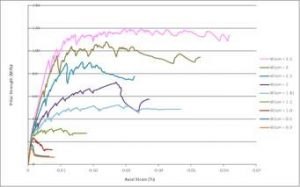The image above represents axial strain.

To compute for axial strain, two essential parameters are needed and these parameters are change in length (ΔL) and length (L).

The formula for calculating the axial strain:

εo = ΔL / L

Where;

εo = Axial Strain
ΔL = Change in Length
L = Total Length

Let’s solve an example;
Calculate the axial strain when the change in length is 18 and total length is 32.

This implies that;

ΔL = Change in Length = 18
L = Total Length = 32

εo = ΔL / L
εo = 18 / 32
εo = 0.5625

Therefore, the axial strain is 0.5625.

Calculating the Change in Length when the Axial Strain and Total Length is Given.

ΔL = εo x L

Where;

ΔL = Change in Length
εo = Axial Strain
L = Total Length

Let’s solve an example;
Calculate the change in length when the axial strain is 21 and total length is 9

This implies that;

εo = Axial Strain = 21
L = Total Length = 9

ΔL = εo x L
ΔL = 21 x 9
ΔL = 189

Therefore, the change in length is 189.

## How to calculate and Solve for Shear Stress | Rock Mechanics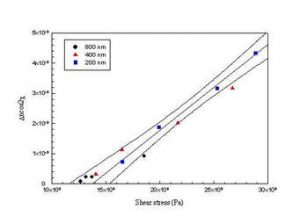The image above represents shear stress.

To compute for shear stress, two essential parameters are needed and these parameters are Shear Force (ΔS) and Area (ΔA).

The formula for calculating the shear stress:

τ = ΔS / ΔA

Where:

τ = Shear Stress
ΔS = Shear Force
ΔA = Area

Let’s solve an example;
Find the shear stress when the shear force is 16 with an area of 30.

This implies that;

ΔS = Shear Force = 16
ΔA = Area = 30

τ = ΔS / ΔA
τ = 16 / 30
τ = 0.53

Therefore, the shear stress is 0.53 Pa.

Calculating the Shear Force when the Shear Stress and Area is Given.

ΔS = τ x ΔA

Where;

ΔS = Shear Force
τ = Shear Stress
ΔA = Area

Let’s solve an example;
Find the shear force with a shear stress of 40 and an area of 12.

This implies that;

τ = Shear Stress = 40
ΔA = Area = 12

ΔS = τ x ΔA
ΔS = 40  x 12
ΔS = 480

Therefore, the Shear Force is 480.

## How to Calculate and Solve for Normal Stress | Rock Mechanics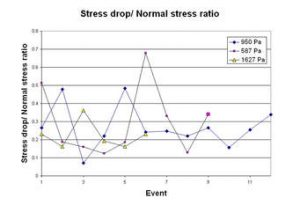The image above represents normal stress.

To calculate for normal stress, two essential parameters are needed and these parameters are normal force (ΔN) and area (ΔA).

The formula for calculating the normal stress:

σn = ΔN / ΔA

Where;

σn = Normal Stress
ΔN = Normal Force
ΔA = Area

Let’s solve an example;
Calculate the normal stress with a normal force of 12 and an area of 22.

This implies that;

ΔN = Normal Force = 12
ΔA = Area = 22

σn = ΔN / ΔA
σn = 12 / 22
σn = 0.54

Therefore, the normal stress is 0.54 Pa.

Calculating the Normal Force when the Normal Stress and Area is Given.

ΔN = σn x ΔA

Where;

ΔN = Normal Force
σn = Normal Stress
ΔA = Area

Let’s solve an example;
Calculate the normal force when the normal stress is 25 with an area of 10.

This implies that;

σn = Normal Stress = 25
ΔA = Area = 10

ΔN = σn x ΔA
ΔN = 25 x 10
ΔN = 250

Therefore, the normal force is 250.

## How to Calculate and Solve for Standing Bubble Point Parameter | The Calculator Encyclopedia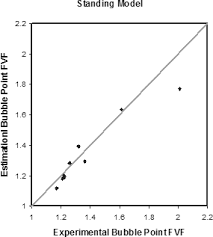The image above represents standing bubble point parameter.

To compute for the standing bubble point parameter, two essential parameters are needed and these parameters are API Gravity (°API) and Temperature (°Rankine) (T).

The formula for calculating standing bubble point parameter:

a = [0.00091(T – 460)] – [0.0125(°API)]

Where:

a = Standing Bubble Point Parameter, a
°API = API Gravity
T = Temperature (°Rankine)

Let’s solve an example;
Find the standing bubble point parameter when API Gravity is 32 and the temperature is 146.

This implies that:

°API = API Gravity = 32
T = Temperature (°Rankine) = 146

a = [0.00091(T – 460)] – [0.0125(°API)]
a = [0.00091(146 – 460)] – [0.0125(32)]
a = [0.00091(-314)] – [0.0125(32)]
a = [-0.28574] – [0.0125(32)]
a = [-0.28574] – [0.4]
a = -0.68574

Therefore, the standing bubble point parameter, a is -0.68574.

## How to Calculate and Solve for MBAL Gas Solubility | The Calculator Encyclopedia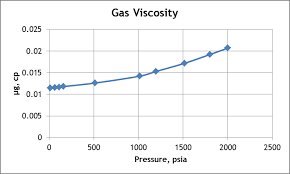The image above represents the MBAL gas solubility.

To compute for the MBAL gas solubility, four essential parameters are needed and these parameters are Specific Gravity of Solution Gas (γg), Specific Gravity of Stock Tank Oil (γo), Oil FVF (Bo) and Oil Density (ρo).

The formula for calculating MBAL gas solubility:

Rs = Boρo – 62.4γo / 0.0136γg

Where:

Rs = MBAL Gas Solubility
γg = Specific Gravity of Solution Gas
γo = Specific Gravity of Stock Tank Oil
Bo = Oil FVF
ρo = Oil Density

Let’s solve an example;
Given that specific gravity of solution gas is 9, specific gravity of stock tank oil is 13, oil FVF is 19 and oil density is 27. Find the MBAL gas solubility?

This implies that;

γg = Specific Gravity of Solution Gas = 9
γo = Specific Gravity of Stock Tank Oil = 13
Bo = Oil FVF = 19
ρo = Oil Density = 27

Rs = Boρo – 62.4γo / 0.0136γg
Rs = 19 x 27 – 62.4 x 13 / 0.0136 x 9
Rs = 513 – 811.19 / 0.1224
Rs = -298.19 / 0.1224
Rs = -2436.27

Therefore, the MBAL gas solubility is -2436.27.

## How to Calculate and Solve for Petrosky-Farshad Gas Solubility Parameter | The Calculator Encyclopedia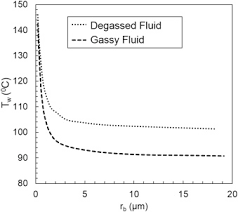The image above represents petrosky-farshad gas solubility parameter.

To compute for the petrosky-farshad gas solubility parameter, two essential parameters are needed and these parameters are API Gravity (°API) and temperature (°Rankine) (T).

The formula for calculating the petrosky-farshad gas solubility parameter:

Rs = [7.716(10-4) (°API)1.541] – [4.561(10-5) (T -460)1.3911]

where:

Rs = Petrosky-Farshad Gas Solubility Parameter, x
°API = API Gravity
T = Temperature (°Rankine)

Let’s solve an example;
Find the petrosky-farshad gas solubility parameter with an API Gravity of 11 and a temperature of 90.

This implies that;

°API = API Gravity = 11
T = Temperature (°Rankine) = 90

Rs = [7.716(10-4) (°API)1.541] – [4.561(10-5) (T -460)1.3911]
Rs = [7.716(10-4) (11)1.541] – [4.561(10-5) (90 – 460)1.3911]
Rs = [7.716(10-4) (11)1.541] – [4.561(10-5) (-370)1.3911]
Rs = [7.716(10-4) (11)1.541] – [4.561(10-5) (NaN)]
Rs = [7.716(10-4) (40.2518)] – [4.561(10-5) (NaN)]
Rs = [0.03105] – [4.561(10-5) (NaN)]
Rs = 0.03105 – NaN
Rs = NaN

Therefore, the Petrosky-Farshad gas solubility parameter, x is NaN.

## How to Calculate and Solve for Petrosky-Farshad Gas Solubility in a Fluid | The Calculator Encyclopedia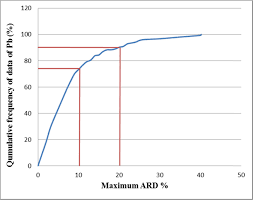The image above represents petrosky-farshad gas solubility.

To compute for the petrosky-farshad gas solubility, three essential parameters are needed and these parameters are gas specific gravity (γg), petrosky-farshad gas solubility parameter (x) and pressure (P).

The formula for calculating the petrosky-farshad gas solubility:

Rs = [(P/112.727 + 12.340)γg0.8439 10x]1.7384

Where:

γg = Gas Specific Gravity
x = Petrosky-Farshad Gas Solubility Parameter
P = Pressure

Let’s solve an example;
Find the petrosky-farshad gas solubility when the gas specific gravity is 16, the petrosky-farshad gas solubility parameter is 22 and the pressure is 80.

This implies that;

γg = Gas Specific Gravity = 16
x = Petrosky-Farshad Gas Solubility Parameter = 22
P = Pressure = 80

Rs = [(P/112.727 + 12.340)γg0.8439 10x]1.7384
Rs = [(80/112.727 + 12.340)160.8439 x 1022]1.9384
Rs = [(0.709 + 12.340)160.8439 x 1022]1.9384
Rs = [(13.049)160.8439 x 1022]1.9384
Rs = [(13.049)10.379 x 1022]1.9384
Rs = [1.354e+24]1.9384
Rs = 8.925e+41

Therefore, the Petrosky-Farshad gas solubility is 8.925e+41.